Next: Mathematical background Up: Analyzing trigonometric equations Previous: Introduction   Contents

Number of roots of trigonometric equation

The purpose of this section is to present an algorithm which enable to determine how many roots has an equation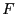in the unknown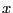of the form: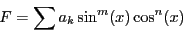(4.1)

with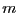inandin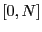, both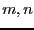being integers, andin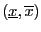.

Subsections

Jean-Pierre Merlet 2012-12-20